This video was brought to you by GeneralPAC.com, making power systems Intuitive, Open and Free for Everyone, Everywhere. Consider subscribing and supporting through patreon.com/GeneralPAC. This is a mechanism for you to support us financially so we can continue making high quality power system video tutorials. Our corporate sponsor for this topic is AllumiaX.com from Seattle, Washington. Contact them for industrial and commercial power system studies.

This is part 3a of the series on fault analysis in power systems where we will discuss three line to ground faults, in other words we will see how three phase currents and voltage quantities are calculated for three line to ground faults, in the previous part we talked about per-unit systems and sequence network diagrams.

In part 3 of this series meaning part 3a, 3b, 3c so forth we will explain the math calculations by hand for current and voltage quantities for various types of faults including the three line to ground, the one line to ground, the line to line and the two line to ground faults. So lets continue with our first example which was introduced in the previous parts and mind you this is gonna be a long tutorial so please bear with us here.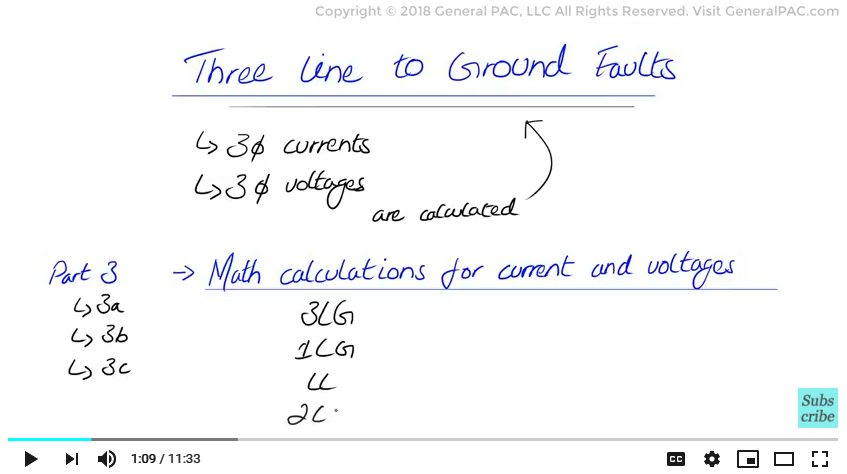We have a three phase synchronous generator having a short circuit capability of 600 MVASc, connected to a 115 kV bus. A delta wye transformer rated at 30 MVA connects the 115 kV bus to the 13.8 kV bus and has a positive and zero sequence impedance of 10 percent at 30 MVA. Step 1 of our calculation asks us to convert the system into per unit values and that is exactly what we did in part 2b of our series, here we draw the same network again.

Next we move to Step 2 which will ask us to identify the type of fault involved, now as mentioned before we have a three line to ground fault which is a symmetrical fault type meaning it’s a balanced fault, now this type of fault only produces positive sequence quantities and does not produce any negative or zero sequence quantities which are currents and voltage values. This is very important for us to keep in mind.

Next we move on to step #3, which asks us to make an un-faulted sequence network from the per unit converted system in step #1, Now these are individual sequence network diagrams which were converted in part 2c of this series and here we draw the same networks below: So we have the positive sequence network and we have the negative sequence network and we have the zero sequence network, again please review part 2c if you like to know more details.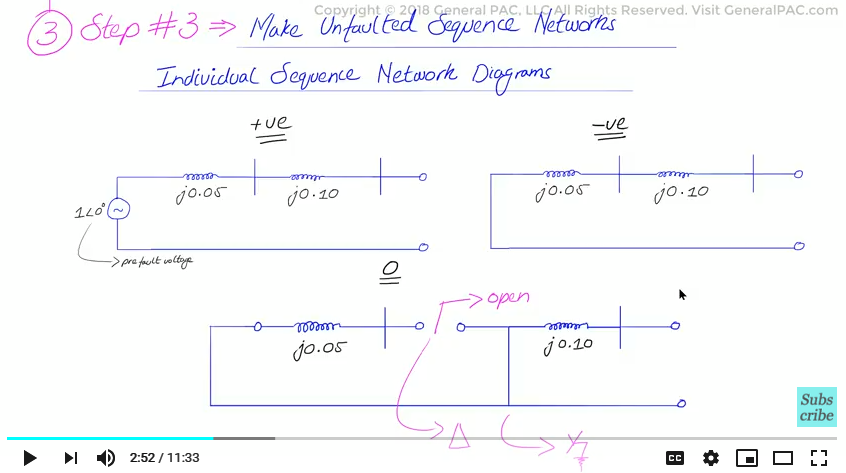And then in step #4, we will draw the faulted sequence network which is interconnected based on the type of fault we have, so given that this is a three line to ground fault which produces only positive sequence currents and voltage quantities we will only have a positive sequence network and that positive sequence network is going to be connected during the faulted condition, now this is pretty trivial here but the diagram shown represents the positive sequence network diagram and we don’t have any other interconnections but this step is important to realize because in the other faults we do interconnect the individual sequence networks.

Now towards step 5 we will hand calculate the sequence network quantities since we have a perfectly balanced three line to ground faults only positive sequence fault currents will be calculated from this faulted network and again this is the positive sequence network diagram so as we analyze the faulted sequence network diagram it’s clear that we simply need to divide the source voltage in per unit values with the total positive sequence impedance which is also in per unit values. The source voltage here is 1 p.u and the sum of series impedance becomes .05 + .1 to be j.15 per unit. After dividing the source voltage with total per unit impedance we get the positive sequence current as j6.67 per unit values. Remember this is complex math with imaginary numbers and although it may not seem important, the negative signs does make a difference here.

Now in step #6, we will calculate the three phase current and voltage values, meaning we need to convert the per unit quantities to phase quantities, This step is going to be long and complicated so understanding the fundamentals of symmetrical components and there is a link in the description section below, is really critical, so let’s continue here. To calculate the base value of current we simply divide the base value of power which is 30 MVA with the voltage on the bus which is 13.8 kV and there is a square root of 3 factor there, now this is very similar to the three phase power formula but solving for the current instead. Now the base value of current is now 1255 amps, now we just simply multiply per unit values that we calculated earlier for the sequence component to the base value that we calculated here and we get 8367 amps at the angle of 90 degrees as the positive sequence current value in ampere quantities so this the positive sequence current values in ampere quantities I repeated that again just to solidify that point. The other two sequence components negative sequence, zero sequence and remember they will be zero in perfectly balanced three line to ground fault.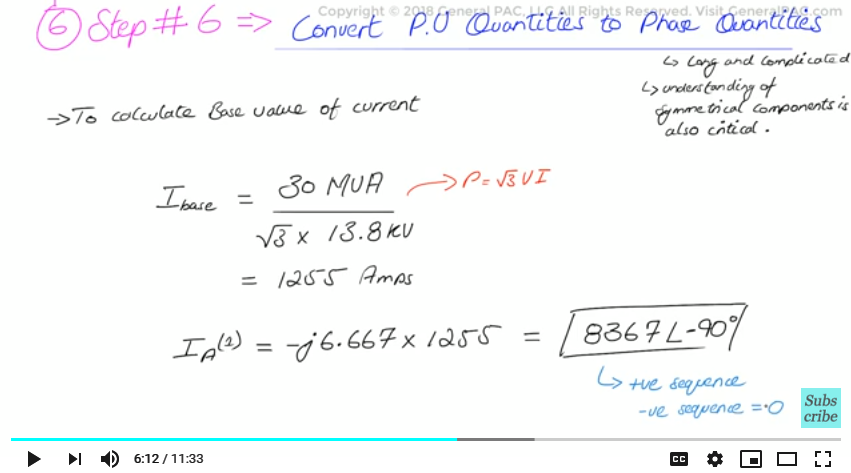Now in step #6 we need to convert the sequence components into phase current quantities, these are currents in amperes that are actually flowing in line a, line b and line c in a three phase power system. To convert to phase current quantities we will use three standard equations that we derived from the phase value sequence components transformation matrix, now this was already discussed in the previous videos in the series on symmetrical components so I am not gonna delve into it too much here. But we quote the three equations as the following:

Now we note here that the negative and zero sequence components are zero because it is a perfectly balanced three phase fault so we simply eliminate those quantities so we can cross them out which simplifies our equations quite a bit for a three phase fault. And here we also have to note that a operator equals the following:

From here all we need to do is just plug in all of the math calculations we did previously so we plug in the values of a and a squared and positive sequence currents of 8367 amps at the angle of -90 degrees in to the three equations and that is gonna give us the current values for phase a, b and c these are the line values so phase a current being 8367 amps at angle -90 degrees, phase b current being 8367 amps at angle 150 degrees and phase c current being 8367 amps at the angle of 30 degrees.

Now to summarize this entire example from step 1 to step 6 we looked at the system and converted it into per unit value and then we took the un-faulted sequence network diagrams from it we drew the positive negative and zero sequence component, we identified the type of fault it was and we eliminated negative and zero sequence components altogether because it was a perfectly balanced three phase fault and then we converted per unit values into sequence components values in amperes and then we converted the sequence component values in amperes to actual phase or line current values for the three phase system, That is the summary of 6 steps.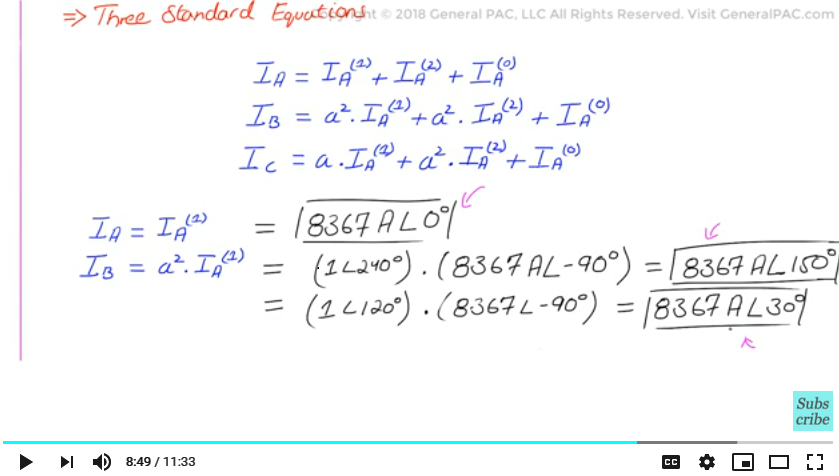Now if we wanted to calculate the voltage quantities, how would we do that, well it’s very simple and it takes the same form as the 6 steps but we will summarize it very quickly below. To calculate the three phase voltage quantities is not very exciting for a perfectly balanced three phase fault because we know that at the point of the fault, the 13.8 kV bus we should expect zero quantities for phase a b and c, voltage quantities and here is how it works mathematically so the positive sequence voltage at the 13.8 kV bus is going to equal 1 at the angle of 0 degrees which is our pre-fault voltage or source voltage minus the total impedance between the source and the point of fault which is j.15 per unit times the current that is flowing through it which is j6.667 per unit now the source voltage minus the voltage drop across the total impedance, if you calculate that out, that simply equals zero per unit, okay so again the negative and zero sequence components are already zero because it is a perfectly balanced system and the positive sequence component at the point of fault on the 13.8 kV bus is also zero as we point out with this calculation

To convert these quantities to phase quantities or phase voltages is really trivial because it is going to equal zero as shown below and remember these are the same matrix as the current quantities we plug in the values and solve for the math, voltage on a b and c on the 13.8 kV bus are simply 0. So that’s the bonus step in between step 6 and step 7.

Now in step 7 we can easily make the current and voltage quantities on the low voltage side of the transformer which is the 13.8 kV side and we can easily reflect that quantity on the high voltage side, however we will do this step in another fault where it is more useful.

So in the next video we will calculate the line to line fault and follow the same methodology. If this video tutorial was useful for you please consider subscribing to generalpac.com or becoming a patron on patreon.com. GeneralPAC.com making power systems intuitive, open and free to everyone, everywhere. Thank you.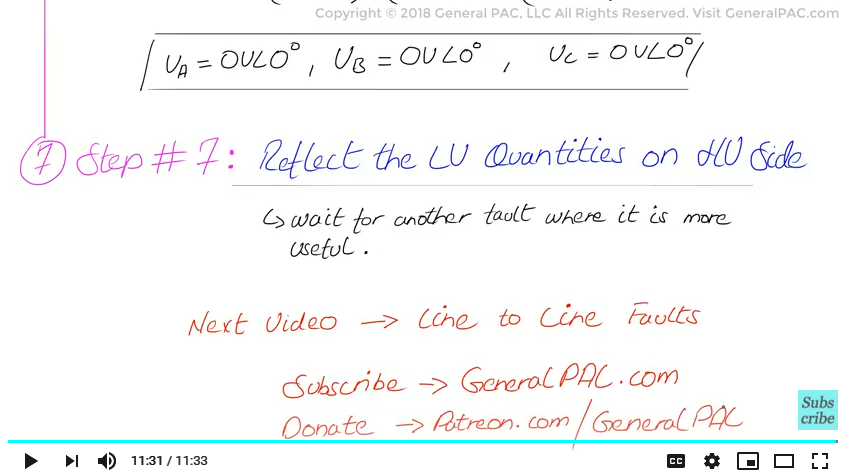#### Greetings from the GeneralPAC Team!

We make high-quality Power Systems Video Tutorials on complex topics that are free and open to everyone!  Thank you so much for supporting us through Patreon so can continue doing good and valuable work.

What is Patreon and why do we use it?

Patreon is a fantastic portal that allows our fans and community to make monthly contribution (like Netflix subscription) so we can continue creating high-quality power systems video tutorials. In return, you get access to incredible perks like voting on future topics, getting your questions answered, access to VIP Q/A webinars with the creators of GeneralPAC, and much more! We THANK YOU for supporting us

Why do we need your support?

An incredible amount of time and effort is needed to develop high-quality video tutorials. Each video (Part 1 for example) takes approximately 10 hours to complete which includes learning the concept ourselves, brainstorming creative ways to teach and explain the concepts, writing the script, audio recording, video recording, and editing. It's no wonder why Hundreds-of-Thousands of people have watched, liked, subscribed, and left positive comments on Youtube channel. Your support truly makes all the difference.